Published:

# Genetic Algorithm

This repository contains simple python implementation of genetic algorithm. There heuristic algorithm searches the space for alternative solutions to the problem in order to find the best solutions.

# Problem

Knapsack problem is one of the most frequently discussed optimization problems. The name of this task comes from the maximization problem of selecting objects so that their total value is as high as possible and at the same time they fit in the backpack.Photo: Dean Pugh

In order to solve a problem we need to generate the data that describes the certain task.

class Task:
def __init__(self, n=None):
self.size = np.random.randint(size, 2 * size)
self.Weight_limit = np.random.randint(10 * self.size, 20 * self.size)
self.Size_limit = np.random.randint(10 * self.size, 20 * self.size)
self.weights = np.random.random(self.n) * 10 * self.Weight_limit / self.n
self.sizes = np.random.random(self.n) * 10 * self.Size_limit / self.n
self.costs = np.random.random(self.n) * self.n


{
'size': 6,
'Weight_limit ': 74,
'Size_limit ': 51,
'weights ': [78.24, 116.79, 27.22, 16.37, 18.49, 105.31],
'sizes': [14.01, 78.47, 34.49, 20.80, 2.29, 41.89],
'costs': [1.72, 0.45, 4.49, 4.85, 4.96, 3.69]
}


# Individual representation

Genetic algorithms take their inspiration from nature, where each animal has its own DNA code. Genetic algorithm tries to create such “animal” that will be the best in given task. To store individual object I’ve created Individual class.

class Individual:
def __init__(self, genome):
self.genome = genome

def mutate(self, mutation_rate):
genome_size = self.genome.shape
no_of_genes_to_change = int(np.ceil(genome_size * mutation_rate))
genes_to_change = random.choices(range(genome_size), k=no_of_genes_to_change)
self.genome[genes_to_change] = -self.genome[genes_to_change] + 1


Genome is binary vector eg. [1,0,1] which tells us that we take item 0 and 2.

# Population

A set of Individuals is nothing else than a Population.

class Population:
def __init__(self, genome_size=None, pop_size=None):
self.population = []
if genome_size != None and pop_size != None:
population_array = np.random.choice([0, 1], size=(pop_size, genome_size))
for genome in population_array:
individual = Individual(genome)
self.population.append(individual)
self.size = len(self.population)


Population is initialized by Individuals with random genome.

# Algorithm

Genetic algorithm is very intuitive due to its straightforward inspiration from nature.

• INITIALIZATION - A certain initial population is drawn.
• SELECTION - The population is evaluated (selection). The best adapted individuals take part in the reproduction process.
• EVOLUTION - Genotypes of selected individuals are subjected to evolutionary operators:
• CROSSOVER - genotypes of new individual are created by combining parental genotypes.
• MUTATION - genotypes are subjected to minor random changes.

This algorithm similar to many other heuristic algorithms is iterative, which means, that its steps are repeated until we achieve satisfying result.

## Initialization

We have discussed it earlier, but one small detail that may be important in implementation is that initialization with equal probability for taking or leaving item may be too much or sometimes to less.

To gain control over initialization we can simply set this probabilities.

population_array = np.random.choice([0, 1], size=(pop_size, genome_size), p=[0.9, 0.1])


## Selection - Tournament

Selection of the individual to reproduce can be done in multiple ways, and choosing the best isn’t always the most effective method.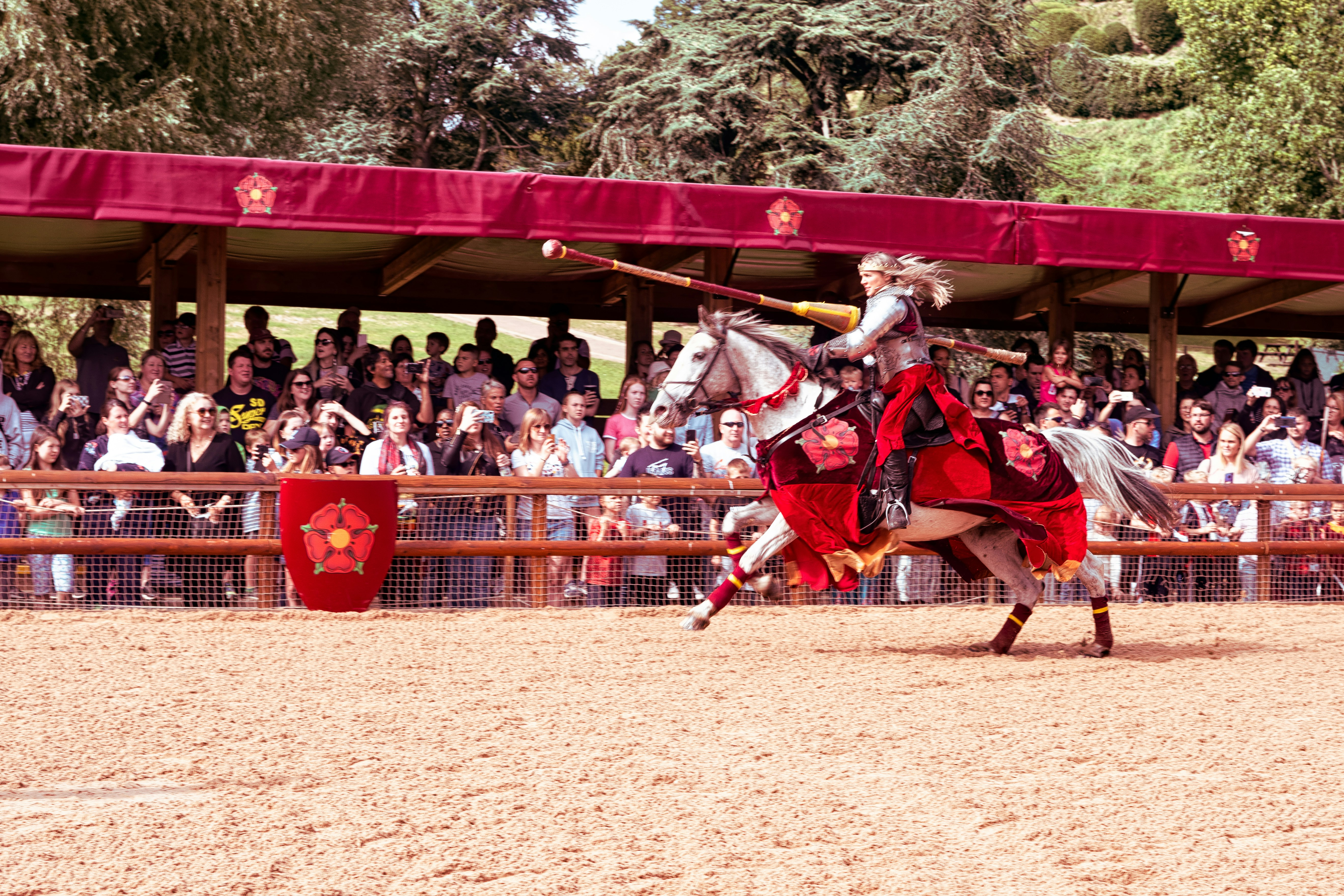Photo: Fas Khan

One of the simplest methods and most effective at once is Tournament Selection. This method implies choosing the best individual from smaller subset of population. This guaranties choosing a strong candidate, but doesn’t implies always choosing the best.

class Population:
selected = random.choices(self.population, k=tournament_size)
evaluation = [elem.evaluate(task) for elem in selected]
idx_best_individual = evaluation.index(max(evaluation))
return selected[idx_best_individual]


tournament_size is a parameter which tells what is the size of a subset of population from which we choose the best individual. You can also spot a evaluate() function. I’ll explain it in following section.

## Evaluation

To see how our individual is preforming we need to check what is the total value that he has gathered, and if the limits are met. If our individual is too greedy and exceed one of the limits (summary weight or size), then its result is penalized by zeroing its score,

class Individual:
return costs_sum
else:
return 0


## Crossover

After we have selected two parents we can make a crossover. In this process a new individual is created. Its genome is a mixture of the parents genome. Our algorithm doesn’t necessarily need to always make crossover to create new individual. The parameter crossover_rate tells us how often new individual should be result of crossover, and how often it can be simply just a copy of one of its parents.

class Population:
def crossover(self, crossover_rate, parent_1, parent_2, task):
if np.random.random() < crossover_rate:
splitting_point = np.random.randint(1, len(parent_1.genome))
result_genome = np.concatenate(
[parent_1.genome[:splitting_point],
parent_2.genome[splitting_point:]]
)
result = Individual(result_genome)
else:
result = parent_1
return result


In this implementation I’ve selected a splitting_point and used it to choose part of the genome that will be inherited from one parent and genome from another.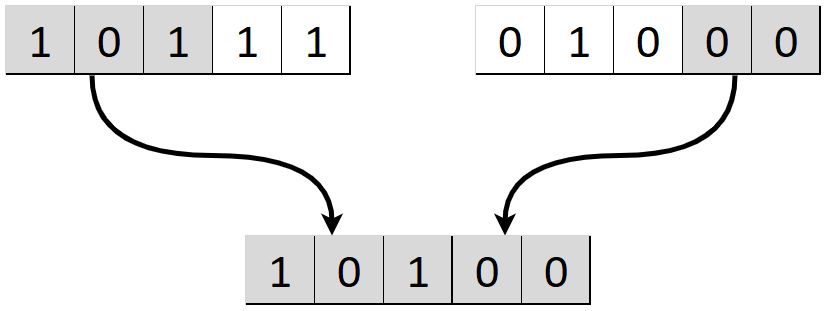## Mutation

This process is just adding a random change to some of the bytes of genome. As in nature the genome of the individual changes through life because of the mutations. This randomness allows our algorithm not to stuck in local optima for too long and find a better solution. The mutation_rate parameter tells how much of the genomes should be changed.

class Individual:
def mutate(self, mutation_rate):
genome_size = self.genome.shape
no_of_genes_to_change = int(np.ceil(genome_size * mutation_rate))
genes_to_change = random.choices(range(genome_size), k=no_of_genes_to_change)
self.genome[genes_to_change] = -self.genome[genes_to_change] + 1


In the last line I’m just changing all selected 0’s to 1’s and otherwise.Photo: museumweek2015

## Genetic algorithm

After we had all the initialization, selection and evolution operators we can construct the code for the whole algorithm. Before we run the algorithm we need to set all the important parameters. in our case we have four of them.

class GeneticAlgorithm:
def __init__(self, populations_size, tournament_size, crossover_rate, mutation_rate):
self.populations_size = populations_size
self.tournament_size = tournament_size
self.crossover_rate = crossover_rate
self.mutation_rate = mutation_rate


Algorithm will be implemented in method fit(). Apart from the task  object we pass a number of iterations that our algorithm will work.

class GeneticAlgorithm:
for _ in range(iterations):
new_population = Population()
for _ in range(population.size):
child = population.crossover(self.crossover_rate, parent_1, parent_2, task)
child.mutate(self.mutation_rate)
population = new_population


# Testing parameters

Great we have our algorithm implemented, let’s test how the value of the parameters affect its performance. To test the performance of algorithm I’ve collected the evaluation of the best individual in population for each iteration. I’ve made 5 different runs of each algorithm to measure the mean and standard deviance for these tests and plotted it on error bar for each value of tested parameter.

## Crossover rate

This parameter tells how often the crossover happens. Low crossover means that we are relying mostly on mutation, and rarely mix the parents to achieve new genome. High value means that we want to create most of the new individuals as a result of crossover.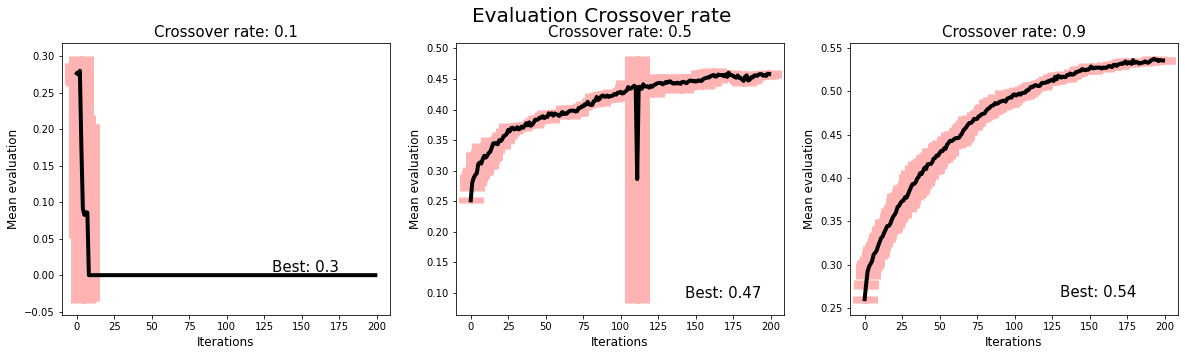Chart above shows that high values of crossover allows algorithm to converge and work more stable. In this example algorithm achieved betted result with crossover 0.9 than 0.5.

## Mutation rate

This parameter describes how much genome elements should be changed to opposite value.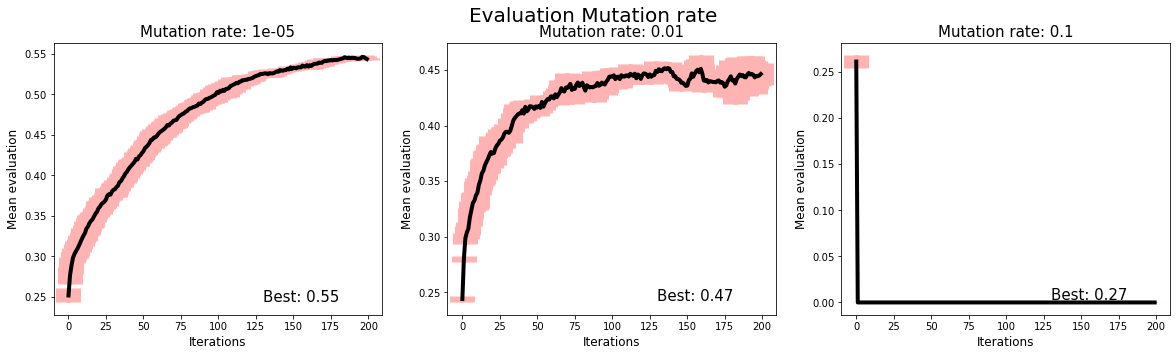As we can see High value of mutation leads our algorithm to 0 very fast. Mutation rate = 0.01 has similar result to 0.0001 but is more noisy and led us to smaller local maximum. The smallest mutation rate the betted but there might be a middle point where mutation is more useful.

## Population size

Size of the population means greater diversity between individuals in each iteration, but high values of this parameter increase the calculation time.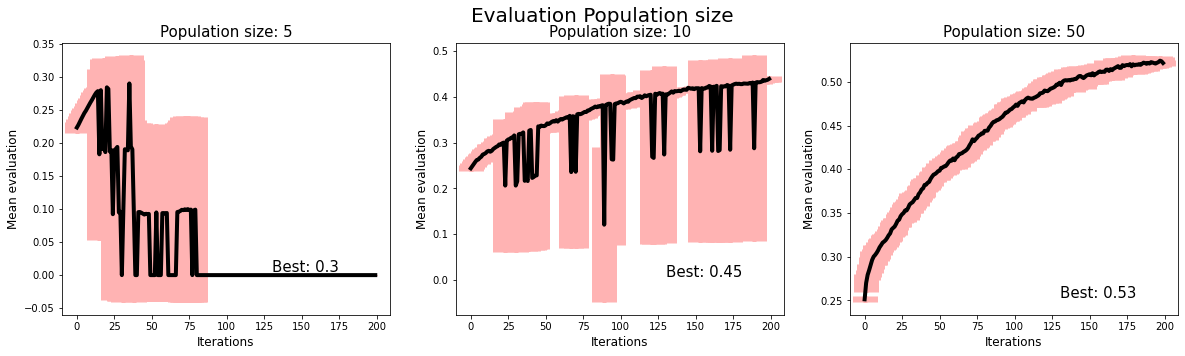Small population died early and didn’t performed much better the random initialization. population size 10 is working better, but still it’s too small and converges slow with lot of anomalies. The highest the population the better, but there is a limit where it only increases the computation time and not the score.

## Tournament size

This parameter tell how much the subset of population will be taken into consideration while selecting a parents for reproduction.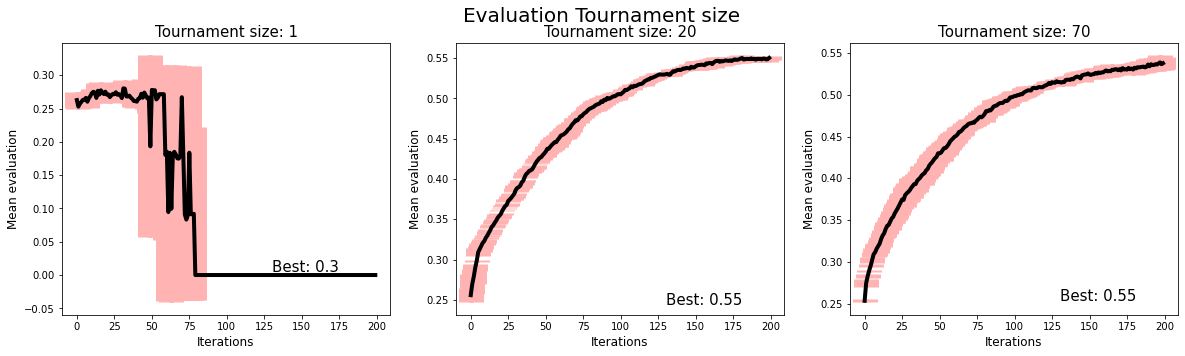Small value is equal to random choose and high value is more likely to just choosing the best individual. This parameter depends on population size, because we choose tournament_size/populations_size*100% of the population, which is relative value.

# Conclusions

Now we all understand why does al these parameters matter, but still there is so much randomness in AG, why is that? The reason for that is easy. Algorithms that are focusing to find the minimum or maximum as in our example tends to stuck in local optima, and role of good algorithm is to tends to global optimum by diverging while in local optimum.

Genetic algorithms have multiple mechanisms to save us from digging in local optima and we can see that in chart comparing mean of population evaluation vs best evaluation. You can see that best is continuously growing, but the mean is oscillation very strong, that means that our algorithm tries to find new solutions, and in almost every iteration this better solution is found.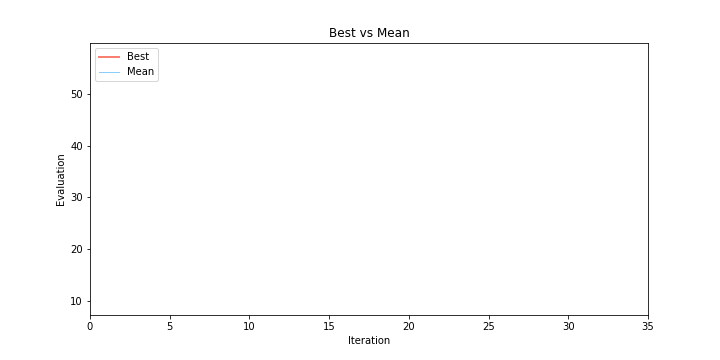# What next?

If you are interested how I have implemented all the other details of the project check out the notebook.

If you are interested in other similar methods check simulated annealing, divide and conquer and other heuristic algorithms.

If you are interested in my other projects, read my blog and LinkedIn.

22 Mar 2020 Mateusz Dorobek

Tags: